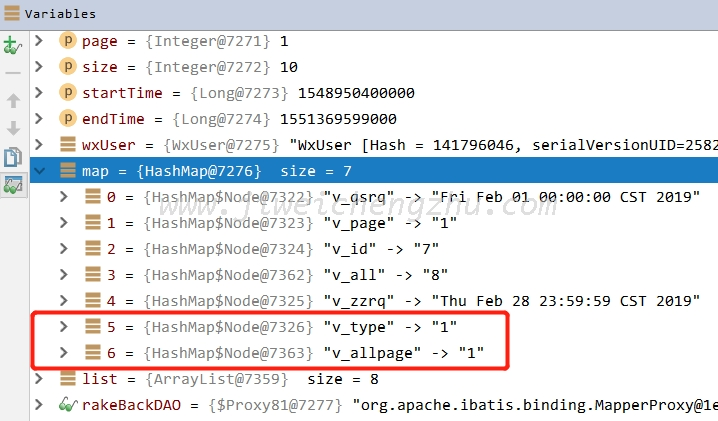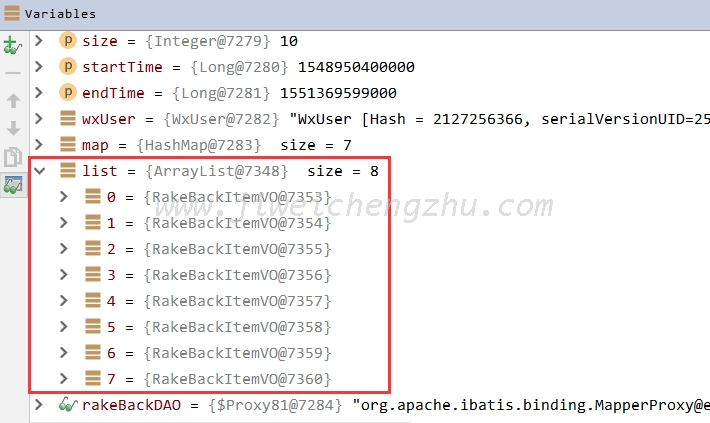# 【原】Mybatis调用MySQL存储过程图文教程

``CREATE DEFINER=`root`@`%` PROCEDURE `query-new`(IN `v_id` int, IN `v_type` int, IN `v_qsrq` date, IN `v_zzrq` date, in v_page int, out v_all int, out v_allpage int)``

## 1、存储过程名字不能用横杠“-”

``### Cause: com.mysql.jdbc.exceptions.jdbc4.MySQLSyntaxErrorException: You have an error in your SQL syntax; check the manual that corresponds to your MySQL server version for the right syntax to use near '-new (``

## 2、out类型参数，必须指定jdbcType

``org.mybatis.spring.MyBatisSystemException: nested exception is org.apache.ibatis.executor.ExecutorException: The JDBC Type must be specified for output parameter.  Parameter: n1``

``````<select id="query_new" statementType="CALLABLE" parameterType="java.util.Map">
call query_new (
#{v_id,mode=IN},
#{v_type,mode=IN},
#{v_qsrq,mode=IN},
#{v_zzrq,mode=IN},
#{v_page,mode=IN},

#{v_all,mode=OUT},
#{v_allpage,mode=OUT}
)
</select>``````

``````<select id="query_new" statementType="CALLABLE" parameterType="java.util.Map">
call query_new (
#{v_id,mode=IN},
#{v_type,mode=IN},
#{v_qsrq,mode=IN},
#{v_zzrq,mode=IN},
#{v_page,mode=IN},

#{v_all,mode=OUT,jdbcType=INTEGER},
#{v_allpage,mode=OUT,jdbcType=INTEGER}
)
</select>``````

``````/**
* 调用存储过程，传入参数、接收参数
*
* @param map 入参，没有封装query，偷个懒，直接用map
*/
void query_new(Map<String, Object> map);``````

debug发现代码没问题，map中的两个out出参也被赋上了值，这个有点儿类似于mybatis的insert上面的keyPropertyId，每次插入新数据之后，会将映射到的javabean的主键ID回写一下。## 3、参数过多，超过指定数量

``Caused by: java.sql.SQLException: Parameter index of 8 is out of range (1, 7)``

``````<select id="query_new" statementType="CALLABLE" parameterType="java.util.Map">
call query_new (
#{v_id,mode=IN,jdbcType=INTEGER},
#{v_type,mode=IN,jdbcType=INTEGER},
#{v_qsrq,mode=IN,jdbcType=TIMESTAMP},
#{v_zzrq,mode=IN,jdbcType=TIMESTAMP},
#{v_page,mode=IN,jdbcType=INTEGER},

#{v_all,mode=OUT,jdbcType=INTEGER},
#{v_allpage,mode=OUT,jdbcType=INTEGER}

#{n1,mode=OUT,jdbcType=TIMESTAMP},
#{n2,mode=OUT,jdbcType=VARCHAR}
#{n3,mode=OUT,jdbcType=DOUBLE},
#{n4,mode=OUT,jdbcType=VARCHAR}
#{n5,mode=OUT,jdbcType=VARCHAR},
#{n6,mode=OUT,jdbcType=VARCHAR}
#{n7,mode=OUT,jdbcType=VARCHAR},
#{n8,mode=OUT,jdbcType=INTEGER}
)
</select>``````

## 4、没有指定返回类型

``````<select id="query_new" statementType="CALLABLE" parameterType="java.util.Map" resultMap="itemMap">
call query_new (
#{v_id,mode=IN,jdbcType=INTEGER},
#{v_type,mode=IN,jdbcType=INTEGER},
#{v_qsrq,mode=IN,jdbcType=TIMESTAMP},
#{v_zzrq,mode=IN,jdbcType=TIMESTAMP},
#{v_page,mode=IN,jdbcType=INTEGER},
#{v_all,mode=OUT,jdbcType=INTEGER},
#{v_allpage,mode=OUT,jdbcType=INTEGER}
)
</select>
<resultMap id="itemMap" type="com.st.money.wx.vo.wx.rake.RakeBackItemVO">
<result column="n1" property="time"/>
<result column="n2" property="source"/>
<result column="n3" property="money"/>
<result column="n4" property="goodsName"/>
<result column="n6" property="status"/>
<result column="n7" property="remark"/>
<result column="n8" property="copperNumber"/>
</resultMap>``````

debug一波，直接报错：

``Caused by: org.apache.ibatis.executor.ExecutorException: A query was run and no Result Maps were found for the Mapped Statement 'com.st.money.wx.dao.RakeBackDAO.query_new'.  It's likely that neither a Result Type nor a Result Map was specified.``

``````/**
* 调用存储过程，传入参数、接收参数
*
* @param map 入参，没有封装query，偷个懒，直接用map
*/
List<RakeBackItemVO> query_new(Map<String, Object> map);``````java调用代码如下：

``````// in入参
Map<String, Object> map = new HashMap<>();
map.put("v_id", userId);
map.put("v_type", moneyType + 1);
map.put("v_qsrq", new Date(startTime));
map.put("v_zzrq", new Date(endTime));
map.put("v_page", page);
// 结果集
List<RakeBackItemVO> list = rakeBackDAO.query_new(map);
// out出参
Integer totalCount = (Integer) map.get("v_all");
Integer totalPage = (Integer) map.get("v_allpage");``````

## 文章评论

请先说点什么
人参与，条评论